## Changing Fractions to Decimals

Fractions may also be written in decimal form (decimal fractions) as either terminating (for example, 0.3) or infinite repeating (for example, 0.66 … ) decimals. To change a fraction to a decimal, simply do what the operation says. In other words,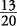means 13 divided by 20. Insert decimal points and zeros accordingly.

Change to decimals.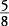So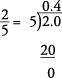So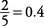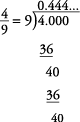Soor